# SAT II Physics : Resistors

## Example Questions

### Example Question #2 : Electricity And Magnetism

There are three resistors in parallel in a circuit with resistances of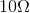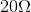, and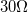.

What is the equivalent resistance?

Possible Answers: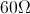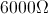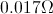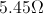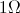Correct answer:Explanation:

The equation for resistors in parallel is: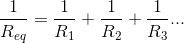We are given the values of the resistors. Using this formula, we can solve for the equivalent resistance.

Plug in the given values and solve.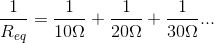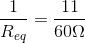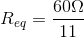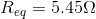### Example Question #1 : Electricity And Magnetism

A voltage ofapplied between the ends of a wire results in a current of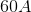. What is the resistance of the wire?

Possible Answers: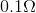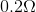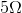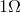Correct answer:Explanation:

This question can be solved using Ohm's law: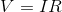We are given the voltage and the current. Using these values, we can solve for the resistance.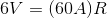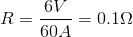### All SAT II Physics Resources